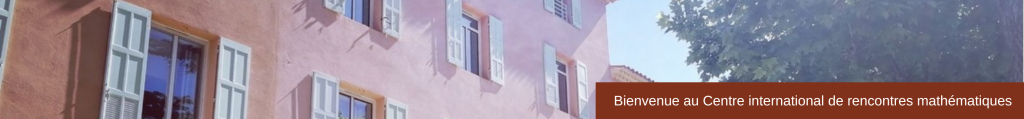## SLIDES

Controllability of Partial Differential Equations and Applications
November 9 -13, 2015

Farid Ammar Khodja (Université de Franche-Comté)
Exact boundary controllability of a system of mixed order with essential spectrum (pdf)

On the optimization of traffic flow at a junction (pdf)

Boris Andreianov (Université de Franche-Comté)
Two results of controllability of hyperbolic conservation laws (pdf)

Claude Bardos (Université Denis Diderot Paris)
Observation of solutions of the Neutron Transport Equation in the diffusion limit (pdf)

Karine Beauchard (ENS Rennes, IRMAR)
Null controllability of degenerate parabolic equations of hypoelliptic type (pdf)

Sébastien Benzekry (Inria Bordeaux-Sud Ouest)
Combined in vivo and in silico quantitative modeling of post-surgery metastatic development and scheduling optimization of anti-cancer therapies (pdf)

Controllability of linear parabolic systems: New phenomena (pdf)

Giuseppe Buttazzo (Universita di Pisa)
Dirichlet-Neumann shape optimization problems (pdf)

Piermarco Cannarsa (University of Rome “Tor Vergata »)
Control and stabilization of degenerate evolution equations in one space dimension (pdf)

Jean-Michel Coron (Université Pierre et Marie Curie)
Rapid stabilization (pdf)

Luz de Teresa (Univ. Nacional Autónoma Mexico)
On hierarchic control for coupled parabolic equations (pdf)

Sylvain Ervedoza (Institut de Mathematiques de Toulouse)
On the stabilization of the incompressible Navier-Stokes equations in a 2d channel with a normal control (pdf)

Florence Hubert (Aix-Marseille Université)
Mathematical modeling of the microtubule dynamic instabilities (pdf)

Olivier Glass (Université Paris-Dauphine)
Control of the movement of a set or particles driven by the stationary Stokes equation (pdf)

Hiroshi Isozaki (University of Tsukuba)
Asymptotic properties of solutions to the elastic wave equation in a half-space (pdf)

Karl Kunisch (University of Graz)
Optimal Control of PDEs with Non-smooth Cost Functionals (pdf)

Gilles Lebeau (Université Nice Sophia Antipolis) &
Spectral inequality and optimal cost of controllability for the Stokes system (pdf)

Arnaud Münch (Université Blaise Pascal – Clermont-Ferrand)
Inverse problems for linear PDEs via variational formulations: Robust numerical approximations (pdf)

Maria Grazia Naso (Università degli Studi di Brescia)
Some results on Timoshenko systems (pdf)

Cristina Pignotti (Universita di L’Aquila)
Stabilization of viscoelastic wave equations with time delay (pdf)

Luc Robbiano (Université Versailles Saint Quentin)
A spectral inequality for the bi-Laplace operator (pdf)

Djamel Eddine Teniou (Université Houari Boumediene, Alger)
Carleman for Stokes-Ventcel (pdf)

Ouahiba Zair (Université Houari Boumediene, Alger)
Some results on null controllability of the heat equation in presence of singularities (pdf)